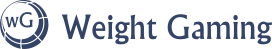# Tried to make a balanced system. Failed. Lets talk about formulas

Here is a level list. You may notice, the level is that of the cake.
You may also notice, how stuff gets out of control by day 10.

The basic idea was, to work out a system for a clicker/idle game, where you have to safely manage the consumption of cake, that is able to level up.
Eventually, this should result in the cake gaining regeneration ability and addictiveness, if “accidentally” mismanaged. 10 “days” until libreCalc tells me to stop is not “eventually”.

Here are some of the forumula used:

Previous day amount eaten, multiplied by lvl of cake of current day: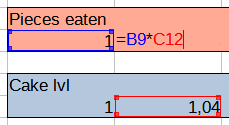Level of cake of the previous day multiplied by amount of cake eaten divided by 25 (basically 1/4th) plus 1: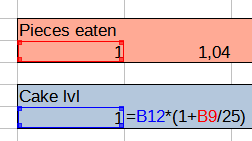Weight is based on some calorie calculator I found: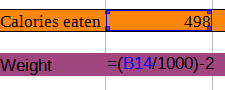Overall, at day 11, you would eat 3 times the calories in 1 gram of uranium (about 18 billion).
Day 12 would have you consume 5000-ish times that.

So, in this discussion, we will be sharing some formulas that are more reasonable and lead to a slow escalation, instead of universal collapse by the end of the month.
Slow but inevidable and looming is the motto here. If it can be prevented, that is fine. If that requires optimal fitness play by week 2 or you are immobile by the end of month 6, that would be PERFECT.

4 Likes

Why not try a percentage based increase rather than adding a multiplied product to it?

Say, based on the efficiency (probably randomized on a 1 to 100 scale) the higher the percentage increase. So, character scored 100% increase, level goes up from one to two. Character scored a 30% increase based on the current level, so it’s 30% of 2.0, which would give a total of 2.15?

Now, to allow for difficulty’s sake, let’s say every single increase you receive points, there’s a penalty for how many times you have to click for a single cake - that way, as time goes on, it’s more difficult to progress through the weight gain.

Say, for example, one devours 1k calories a day. Every calorie = 1 calorie obtained. Let’s say one devours 5k. Every calorie obtains a smaller amount.

I don’t know. I’m not a math whiz, I just wanted to contribute to the conversation…

1 Like

You could use a curve fitting model make whatever growth rate you want to happen. Lets say for example you want the average player to be 1000lbs by month 6 (180 days) and they start at 100lbs. You could use a curve fitting website such as https://mycurvefit.com/ to plot a curve that has has day 0: 100lbs, day 180: 1000lbs and maybe like day 60: 200lbs.

If you fit that data to a quadratic regression, it will have a nice exponential curve that steadily gets faster as time goes on but doesn’t spiral out of control instantly (though given that it exponential if you go much beyond 180 days you are likely to spiral out of control with a quadratic formula.)

Here a quick example I made on that myCurveFit website. You could play around with using different points and fitting methods to get different results.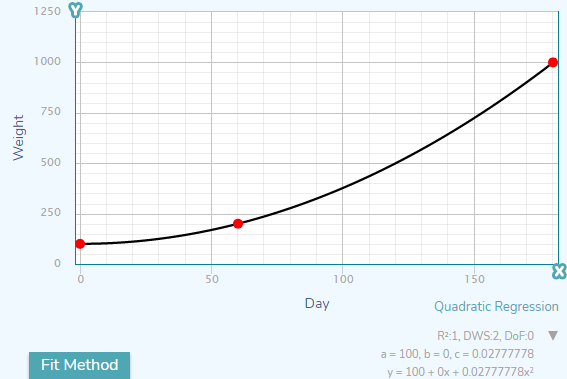2 Likes

Looks like some weirdness going on in your calorie calculation. Also what unit is (Calories eaten/1000)-2 supposed to produce? As far as I’m aware there’s about 3300 calories in a pound of fat.

well for realism your weight gain equation should be something like

Fat_Weight_gain = ( Calories_consumed - Base_metabolic_rate - Calories_burned_via_exercise ) / 3300

Where base metabolic rate is:

I’d strongly suggest testing any formulas with a graphing calculator. The default windows calculator can do this. Anything that shoots off to infinity is not going to be your friend (ie. anything that repeatedly multiplies a value is going to do this). Find things that rise but have an upper limit.

Likely the simplest is y = x / (x + c) for positive x.